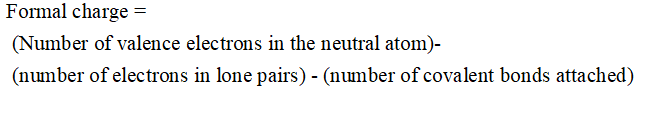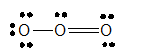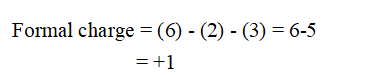# Calculate the formal charge on the indicated atom in eachof the following molecules or ions: (a) the central oxygenatom in O3, (b) phosphorus in PF6-, (c) nitrogen in NO2,(d) iodine in ICl3, (e) chlorine in HClO4 (hydrogen is bondedto O).

Question
1 views

Calculate the formal charge on the indicated atom in each
of the following molecules or ions: (a) the central oxygen
atom in O3, (b) phosphorus in PF6-, (c) nitrogen in NO2,
(d) iodine in ICl3, (e) chlorine in HClO4 (hydrogen is bonded
to O).

check_circle

Step 1

The formal charge on the indicated atom in each
of the following molecules or ions is to be calculated.

(a) The central oxygen atom in O3,

(b) Phosphorus in PF6-,

(c) Nitrogen in NO2,
(d) Iodine in ICl3,

(e) Chlorine in HClO4 (hydrogen is bonded to O).

Step 2

Formal charge is the actual charge on individual atoms of a molecule or compound.

• The sum of formal charges on each atom is always equal to the total charge of the molecule or ion.
• The formula used to calculate formal charge is :Step 3
1. The central oxygen atom in O3:

The Lewis structure of O3 is:Now, the central atom Oxygen has 6 valence electrons.

Also,

Number of electrons in lone pairs around central O atom = 2

Number of bonds around central O atom = 3

Therefore, formal charge can be calculated as:Hence, the formal charge on the central oxygen atom in O3 is +1.

...

### Want to see the full answer?

See Solution

#### Want to see this answer and more?

Solutions are written by subject experts who are available 24/7. Questions are typically answered within 1 hour.*

See Solution
*Response times may vary by subject and question.
Tagged in

### Chemistry